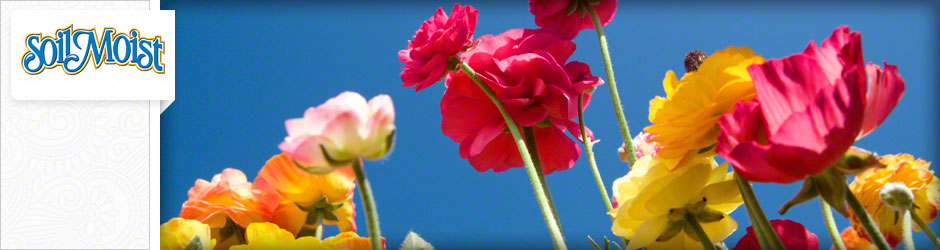Soil Moist Product Calculators

JRM Soil Moist Granular Usage Calculator by:

Caliper Size

Enter the number of calipers:

Amount of Granular (oz.) needed:

Boxed Trees

Enter the number of trees:

Amount of Granular (oz.) needed:

Foot of Rootball

Enter the number of rootballs:

Amount of Granular (oz.) needed:

Container Size

Enter the number of container:

Amount of Granular (tsp or oz.) needed:

Cubic Foot of Soil

Enter your cubic feet of soil:

Amount of Plus (oz.) needed:

Cubic Yards of Soil

Enter your cubic yards of soil:

Amount of Granular (lbs.) needed:

JRM Soil Moist Plus Usage Calculator by

Caliper Size

Enter the number of calipers:

Amount of Plus (oz.) needed:

Boxed Trees

Enter the number of trees:

Amount of Plus (oz.) needed:

Container Size

Enter the number of container:

Amount of Plus (tsp or oz.) needed:

Cubic Foot of Soil

Enter your cubic feet of soil:

Amount of Plus (oz.) needed:

Cubic Yards of Soil

Enter your cubic yards of soil:

Amount of Granular (lbs.) needed:

JRM Transplant Usage Calculator by

Container Size

Enter the number of containers:

Amount of Transplant (oz.) needed:

Plant Height

Enter the number of plants:

Amount of Transplant (oz.) needed:

Caliper Size

Enter the number of calipers:

Amount of Transplant (oz.) needed:

Box Size

Enter the number of boxes:

Amount of Transplant (oz.) needed:

JRM Soil Moist Mats Usage Calculator by

Container Size

Enter the number of containers:

Number of Soil Moist Mats needed:

JRM Soil Moist Paks & Paks Plus Usage Calculator by

Container Size

Enter the number of containers:

Number of Paks needed:

Caliper Size

Enter the number of calipers:

Number of Paks needed:

JRM Ecto Tabs Usage Calculator by

Plant Height

Enter the number of plants:

Number of Tabs needed:

Caliper Inch of Tree

Enter the number of calipers:

Number of Tabs needed:

JRM Plant Tabs Usage Calculator by

Plant Height

Enter the number of plants:

Number of Tabs needed:

Caliper Inch of Tree

Enter the number of calipers:

Number of Tabs needed:

JRM Soil Moist Flower Bed Usage Calculator by

Container Size

Enter the number of containers:

Amount of Plus (oz.) needed:

Caliper Size

Enter the number of calipers:

Amount of Plus (oz.) needed:

Flower Beds

Enter the number of flower beds:

Amount of Plus (lbs.) needed: